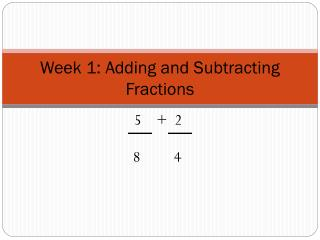DownloadDownload PresentationWeek 1: Adding and Subtracting Fractions

# Week 1: Adding and Subtracting Fractions

Download Presentation## Week 1: Adding and Subtracting Fractions

- - - - - - - - - - - - - - - - - - - - - - - - - - - E N D - - - - - - - - - - - - - - - - - - - - - - - - - - -
##### Presentation Transcript

1. Week 1: Adding and Subtracting Fractions 5 + 2 8 4

2. WITHOU T A CALCULATOR!!!!!!

3. Week 1 Adding and Subtracting Fractions • Multiply the numerators by the opposite denominator • Multiply the 2 denominators to find common factor (NEW DENOMIANTOR for both fractions!) • Add or subtract the numerators • Keep the same denominator • Reduce your fractions – without a calculator!!!!!! 5 + 2 8 4

4. Multiply the numerators by the opposite denominator • Multiply the 2 denominators to find common factor (NEW DENOMIANTOR for both fractions!) • Add or subtract the numerators • Keep the same denominator • Reduce your fractions – without a calculator!!!!!! 9 _ 2 12 4

5. Multiply the numerators by the opposite denominator • Multiply the 2 denominators to find common factor (NEW DENOMIANTOR for both fractions!) • Add or subtract the numerators • Keep the same denominator • Reduce your fractions – without a calculator!!!!!! 3 + 3 4 7